The EXPAND Procedure

The OUTEST= data set contains the coefficients of the spline curves fit to the input series. The OUTEST= data set is of interest if you want to verify the interpolating curve PROC EXPAND uses, or if you want to use this function in another context, (for example, in a SAS/IML program).

The OUTEST= data set contains the following variables:

• the BY variables, if any

• VARNAME, a character variable containing the name of the input variable to which the coefficients apply

• METHOD, a character variable containing the value of the METHOD= option used to fit the series

• OBSERVED, a character variable containing the first letter of the OBSERVED= option name for the input series

• the ID variable that contains the lower breakpoint (or "knot") of the spline segment to which the coefficients apply. The ID variable has the same name as the variable used in the ID statement. If an ID statement is not used, but the FROM= option is used, then the name of the ID variable is DATE or DATETIME, depending on whether the FROM= option indicates SAS date or SAS datetime values. If neither an ID statement nor the FROM= option is used, the ID variable is named TIME.

• CONSTANT, the constant coefficient for the spline segment

• LINEAR, the linear coefficient for the spline segment

• CUBIC, the cubic coefficient for the spline segment

For each BY group, the OUTEST= data set contains observations for each polynomial segment of the spline curve fit to each input series. To obtain the observations defining the spline curve used for a series, select the observations where the value of VARNAME equals the name of the series.

The observations for a series in the OUTEST= data set encode the spline function fit to the series as follows. Let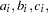and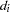be the values of the variables CUBIC, QUAD, LINEAR, and CONSTANT, respectively, for the i th observation for the series. Let
be the value of the ID variable for the i th observation for the series. Let n be the number of observations in the OUTEST= data set for the series. The value of the spline function evaluated at a point x is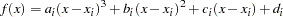where the segment number i is selected as follows: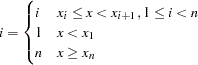In other words, if x is between the first and last ID values (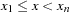), use the observation from the OUTEST= data set with the largest ID value less than or equal to x. If x is less than the first ID value
, then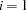. If x is greater than or equal to the last ID value (
), then
.

For METHOD=JOIN, the curve is a linear spline, and the values of CUBIC and QUAD are 0. For METHOD=STEP, the curve is a constant spline, and the values of CUBIC, QUAD, and LINEAR are 0. For METHOD=AGGREGATE, no coefficients are output.Previous Page | Next Page | Top of Page